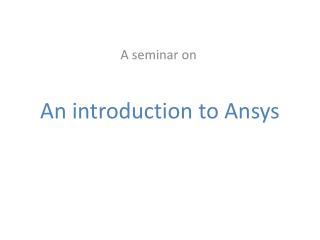DownloadDownload PresentationAn introduction to Ansys

# An introduction to Ansys

Download Presentation## An introduction to Ansys

- - - - - - - - - - - - - - - - - - - - - - - - - - - E N D - - - - - - - - - - - - - - - - - - - - - - - - - - -
##### Presentation Transcript

1. An introduction to Ansys A seminar on

2. Content • Introduction • A simple example • Steps involved • Brief explanation of element types • Analysis in ansys • Summary

3. Introduction to Ansys • It is an engineering simulation software developed in 1970 by  Dr. John A. Swanson • It was developed to use finite element analysis to solve structural problems. • At present it is a leading software which can be used for structural, thermal, electrical, fluid problems, as well as to simulate biological components.

4. An example of simple cylinder under tensile loading. Material: Aluminium Yield stress: 11 Mpa Young’s Modulus: 70 Gpa Poisson’s ratio: 0.35 Diameter= 20 mm Length= 100 mm

5. Stress induced due to gradual tensile load of 3000 N on Z direction is • Stress = 3000/314.28 = 9.54 Mpa e = stress/E = 9.54/70000 dl = L*e = 9.54x100/70000 = 0.013628 mm

6. Steps involved • Creation of CAD model • Selection of suitable element type. • Selecting suitable material properties. • Mesh generation • Defining boundary conditions • Generate a solution • Analyze results

7. Element types • Link element It is a simple element which takes uniaxial loading that is compression or tension. It has 2 nodes per element, with 2 degrees of freedom per node. Ux and Uy

8. 2. Beam element is a uniaxial element with tension, compression, and bending capabilities. It has 2 nodes per element, with 6 degree of freedom per node. Ux, Uy, Ux, Rot x, Rot y, Rot z 3. Pipe element Similar to beam element but with tension, compression, torsion and bending capabilities

9. 4. Solid This element is used to mesh a 3D model. Its subtypes are quadrilateral and tetrahedral. Quadrilateral 4 node 8 node Tetrahedral 4 Node 10 Node

10. Brick 8 node 20 node

11. Material properties 1. Linear Elastic Isotropic Orthotropic Anisotropic 2. Non-linear Elastic Inelastic Viscoelastic 3. Density 4. Thermal expansion 5. Damping 6. Friction coefficient

12. Mesh generation • Discritizing the model into number of elements is called as meshing. • Validity of analysis greatly depends on meshing. • Finer mesh >> more accurate results >> more computational time

13. 3D model in Ansys

14. Meshed model with coarse mesh

15. Meshed model with fine mesh

16. Model meshed with Tet element

17. Modeling of cylinder • Element selected Link • Material properties • Poisson’s ratio 0.35 • Young’s modulus 70000 Mpa • Force applied 3000 N

18. Nodes in model of cylinder

19. Displacement result

20. Displacement using beam element

21. Model of cylinder

22. Coarse mesh with boundary conditions

23. Displacement

24. Stress

25. Fine mesh

26. Displacements

27. Summary • Brief steps in finite element analysis using Ansys • Introduction to Ansys, various element types. • Comparison between exact results and results obtained using FEA.

28. References • M. A. Maleque and M. S. Salit, “Materials Selection and Design”, SpringerBriefs in Materials, DOI: 10.1007/978-981-4560-38-2_2. • A.E. Tekkaya, P.A.F. Martins, (2009) "Accuracy, reliability and validity of finite element analysis in metal forming: a user's perspective", Engineering Computations, Vol. 26 Iss: 8. • Ansysmodeling and meshing guide. • http://mostreal.sk/html/elem_55/chapter4/ • http://web.mit.edu/calculix_v2.4/CalculiX/ccx_2.4/doc/ccx/

29. Thank you